# Doc: Some functions and their Graphs JEE Notes | EduRev

## JEE : Doc: Some functions and their Graphs JEE Notes | EduRev

The document Doc: Some functions and their Graphs JEE Notes | EduRev is a part of the JEE Course Mathematics (Maths) Class 11.
All you need of JEE at this link: JEE

Different types of functions and their graphical representation.

Identity function

A function f:R → R is said to be an identity function if f(x) = x, ∀ x ϵ R denoted by IR.

Let A = {1, 2, 3}

The function f: A → A defined by f(x) = x is an identity function.

f = {(1,1), (2,2), (3,3)}.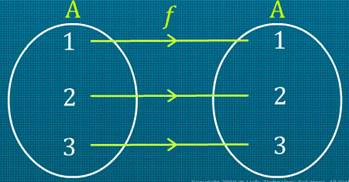The graph of an identity function is a straight line passing through the origin.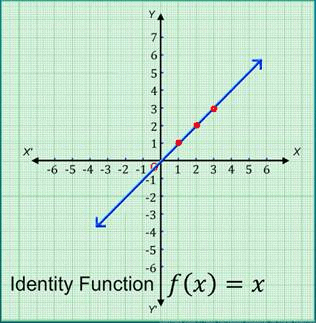Each point on this line is equidistant from the coordinate axes.

The straight line makes an angle of 45° with the coordinate axes.

Linear function
A function f:R → R is said to be a linear function if f (x) = ax + b, where a ≠ 0, a and b are real constants, and x is a real variable.

Consider the linear function, f(x) = 3x + 7, ∀ x ϵ R

The ordered pairs satisfying the linear function are (0, 7), (-1, 4), (-2, 1).

On plotting these points on the Cartesian plane and then joining them, we get the graph of the linear function f of x is equal to 3x + 7, ∀ x ϵ R as shown in the figure.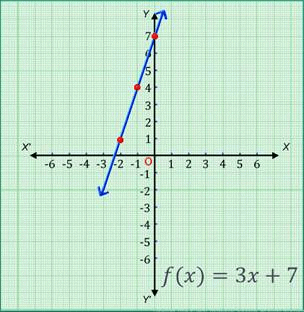Constant function
A function f:R → R is said to be a constant function, if f(x) = c , ∀ x ϵ R, where c is a constant.

Let f:R → R be a constant function defined by f(x) = 4 , ∀ x ϵ R.

The ordered pairs satisfying the linear function are: (0, 4), (-1, 4), (2, 4).

If the range of a function is a singleton set, then it is known as a constant function.

On plotting these points on the Cartesian plane and then joining them, we get the graph of the constant function f of x = 4,∀ x ϵ R as shown.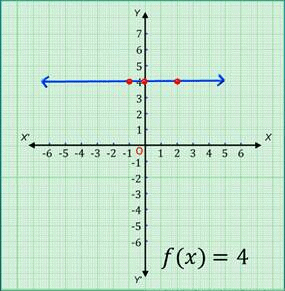Also, from the graph, we can conclude that the graph of a constant function, f(x) = c, is always a straight line parallel to the X-axis, intersecting the Y-axis at (0, c).

Polynomial function
A function f:R → R is said to be a polynomial function if for all x in R, y = f(x) = a0+ a1x + a2x2 + ……………..+ anxn, where n is a non-negative integer and a0, a1, a2…….. an are real numbers.

Examples of polynomial functions

f(x) = x2 + 5x + 6 ,∀ x ϵ R

and f (x) = x3 + 4x + 2 ,∀ x ϵ R

Note: In a polynomial function, the powers of the variables should be non-negative integers.For example, f(x) = √x + 2 (∀ x ϵ R) is not a polynomial function because the power of x is a rational number.

Consider the polynomial function, f(x) = 3x2 +2x -3 ,∀ x ϵ RThe ordered pairs satisfying the polynomial function are (0, -3), (-1, -2), (1, 2), (2, 13), (-2, 5).

On plotting these points on the Cartesian plane and then joining them, we get the graph of the polynomial function f of x is equal to 3 x2 + 2 x - 3 ,∀ x ϵ R as shown.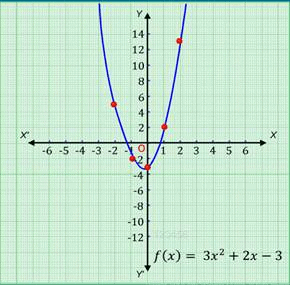Rational function
If f(x) and g(x) be two polynomial functions, then f(x)/g(x) such that g(x) ≠ 0 and ∀ x ϵ R, is known as a rational function.

Let us consider the function f ( x) = 2x -5/3x - 2 (x ≠ ⅔).

The ordered pairs satisfying the polynomial function are: (0, 5), (2, -¼), (1, -3).

On plotting these points on the Cartesian plane and then joining them, we get the graph of the given rational function as shown.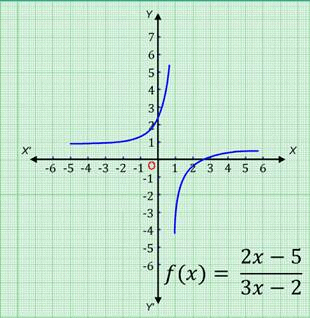Modulus function
A function f: R → R defined by f(x) = |x| (∀ x ϵ R) is known as a modulus function.

If x is negative, then the value of the function is minus x, and if x is non-negative, then the value of the function is x. i.e. f(x) = x if x ≥ 0 = - x if x < 0.

The ordered pairs satisfying the polynomial function are (0, 0), (-1, 1), (1, 1), (-3, 3), (3, 3).

On plotting these points on the Cartesian plane and then joining them, we get the graph of modulus function f of x is equal to mod of x.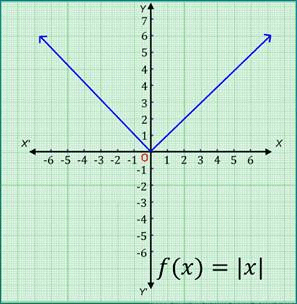Greatest integer function
A function f: R → R defined by f(x) = [x],∀ x ϵ R assumes the value of the greatest integer, less than or equal to x.

From the definition of [x], we can see that

[x] = -1 for -1 £ x < 0

[x] = 0 for 0 £ x < 1

[x] = 1 for 1 £ x < 2

[x] = 2 for 2 £ x < 3, and so on.

⇒  f(2.5) will give the value 2 and f(1.2) will give the value 1, and so on…

Hence, the graph of the greatest integer function is as shown.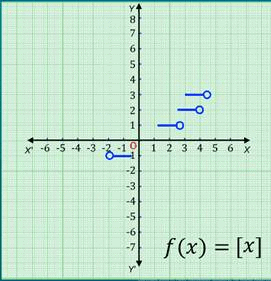Signum Function

A function f: R→ R defined by

f(x) = { 1, if x > 0; 0, if x = 0; -1, if x < 0

Signum or the sign function extracts the sign of the real number and is also known as step function.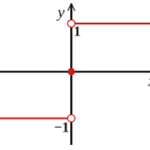Algebra of Real Functions

Real-valued Mathematical Functions

In mathematics, a real-valued function is a function whose values are real numbers. It is a function that maps a real number to each member of its domain. Also, we can say that a real-valued function is a function whose outputs are real numbers i.e., f: R→R (R stands for Real).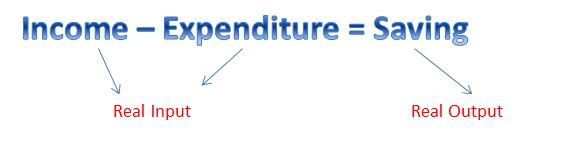Algebra of Real Functions
In this section, we will get to know about addition, subtraction, multiplication, and division of real mathematical functions with another.

Let f and g be two real valued functions such that f: X→R and g: X→R where X ⊂ R. The addition of these two functions (f + g): X→R  is defined by:

(f + g) (x) = f(x) + g(x), for all x ∈ X.

Subtraction of One Real Function from the Other
Let f: X→R and g: X→R be two real functions where X ⊂ R. The subtraction of these two functions (f – g): X→R  is defined by:

(f – g) (x) = f(x) – g(x), for all x ∈ X.

Multiplication by a Scalar

Let f: X→R be a real-valued function and γ be any scalar (real number). Then the product of a real function by a scalar γf: X→R is given by:

(γf) (x) = γ f(x), for all x ∈ X.

Multiplication of Two Real Functions

The product of two real functions say, f and g such that f: X→R and g: X→R, is given by

(fg) (x) = f(x) g(x), for all x ∈ X.

Division of Two Real Functions

Let f and g be two real-valued functions such that f: X→R and g: X→R where X ⊂ R. The quotient of these two functions (f  ⁄ g): X→R  is defined by:

(f / g) (x) = f(x) / g(x), for all x ∈ X.

Note: It is also called point wise multiplication.

Solved Example for You

Problem: Let f(x) = x3 and g(x) = 3x + 1 and a scalar, γ= 6. Find

1. (f + g) (x)

2. (f – g) (x)

3. (γf) (x)

4. (γg) (x)

5. (fg) (x)

6. (f / g) (x)

Solution:
We have,

1. (f + g) (x) = f(x) + g(x) = x+ 3x + 1.

2. (f – g) (x) = f(x) – g(x) = x– (3x + 1) = x– 3x – 1.

3. (γf) (x) = γ f(x) = 6x

4. (γg) (x) = γ g(x) = 6 (3x + 1) = 18x + 6.

5. (fg) (x) = f(x) g(x) = x(3x +1) = 3x4 + x3.

6. (f / g) (x) = f(x) / g(x) = x/ (3x +1), provided x ≠ – 1/3.

Offer running on EduRev: Apply code STAYHOME200 to get INR 200 off on our premium plan EduRev Infinity!

## Mathematics (Maths) Class 11

158 videos|179 docs|161 tests

,

,

,

,

,

,

,

,

,

,

,

,

,

,

,

,

,

,

,

,

,

;